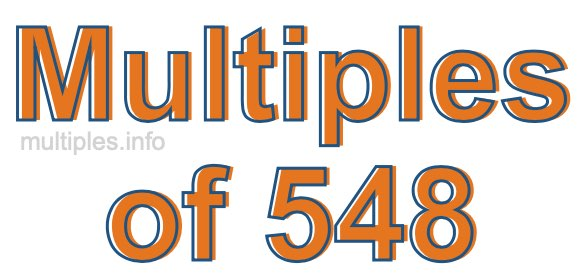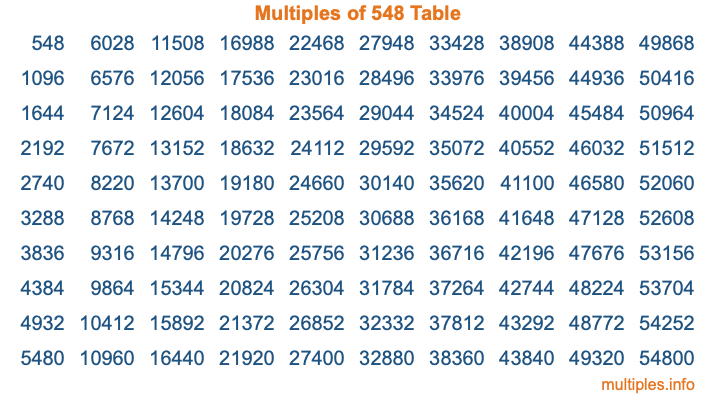Multiples of 548Welcome to the Multiples of 548 page. Here we will first teach you everything you will ever need to know about the multiples of 548, and then give you a study guide summary of everything we taught you to make sure you remember it all. Use this page to look up facts and learn information about the multiples of 548. This page will make you a multiples of five hundred forty-eight expert!

Definition of Multiples of 548
Multiples of 548 are all the numbers that when divided by 548 equal an integer. Each of the multiples of 548 are called a multiple. A multiple of 548 is created by multiplying 548 by an integer.

Therefore, to create a list of multiples of 548, you start with 1 multiplied by 548, then 2 multiplied by 548, then 3 multiplied by 548, and so on for as long as you want. Thus, the list of the first five multiples of 548 is 548, 1096, 1644, 2192, and 2740. To see a larger list of multiples of 548, see the printable image of Multiples of 548 further down on this page. We also have a category where you can choose any nth multiple of 548.

Multiples of 548 Checker
The Multiples of 548 Checker below checks to see if any number of your choice is a multiple of 548. In other words, it checks to see if there is any number (integer) that when multiplied by 548 will equal your number. To do that, we divide your number by 548. If the the quotient is an integer, then your number is a multiple of 548.

Is  a multiple of 548?

Least Common Multiple of 548 and ...
A Least Common Multiple (LCM) is the lowest multiple that two or more numbers have in common. This is also called the smallest common multiple or lowest common multiple and is useful to know when you are adding our subtracting fractions. Enter one or more numbers below (548 is already entered) to find the LCM.

Check out our LCM Calculator if you need more details about the Least Common Multiple or if you need the LCM for different numbers for adding and subtraction fractions.

nth Multiple of 548
As we stated above, 548 is the first multiple of 548, 1096 is the second multiple of 548, 1644 is the third multiple of 548, and so on. Enter a number below to find the nth multiple of 548.

th multiple of 548

Multiples of 548 vs Factors of 548
548 is a multiple of 548 and a factor of 548, but that is where the similarities end. All postive multiples of 548 are 548 or greater than 548. All positive factors of 548 are 548 or less than 548.

Below is the beginning list of multiples of 548 and the factors of 548 so you can compare:

Multiples of 548: 548, 1096, 1644, 2192, 2740, etc.

Factors of 548: 1, 2, 4, 137, 274, 548

As you can see, the multiples of 548 are all the numbers that you can divide by 548 to get a whole number. The factors of 548, on the other hand, are all the whole numbers that you can multiply by another whole number to get 548.

It's also interesting to note that if a number (x) is a factor of 548, then 548 will also be a multiple of that number (x).

Multiples of 548 vs Divisors of 548
The divisors of 548 are all the integers that 548 can be divided by evenly. Below is a list of the divisors of 548.

Divisors of 548: 1, 2, 4, 137, 274, 548

The interesting thing to note here is that if you take any multiple of 548 and divide it by a divisor of 548, you will see that the quotient is an integer.

Multiples of 548 Table
Below is an image of the first 100 multiples of 548 in a table. The table is in chronological order, column by column. The first column has the first ten multiples of 548, the second column has the next ten multiples of 548, and so on.The Multiples of 548 Table is also referred to as the 548 Times Table or Times Table of 548. You are welcome to print out our table for your studies.

Negative Multiples of 548
Although not often discussed or needed in math, it is worth mentioning that you can make a list of negative multiples of 548 by multiplying 548 by -1, then by -2, then by -3, and so on, to get the following list of negative multiples of 548:

-548, -1096, -1644, -2192, -2740, etc.

Multiples of 548 Summary
Below is a summary of important Multiples of 548 facts that we have discussed on this page. To retain the knowledge on this page, we recommend that you read through the summary and explain to yourself or a study partner why they hold true.

There are an infinite number of multiples of 548.

A multiple of 548 divided by 548 will equal a whole number.

548 divided by a factor of 548 equals a divisor of 548.

The nth multiple of 548 is n times 548.

The largest factor of 548 is equal to the first positive multiple of 548.

548 is a multiple of every factor of 548.

548 is a multiple of 548.

A multiple of 548 divided by a divisor of 548 equals an integer.

548 divided by a divisor of 548 equals a factor of 548.

Any integer times 548 will equal a multiple of 548.

Multiples of a Number
Here you can get the multiples of another number, all with the same attention to detail as we did for multiples of 548 on this page.

Multiples of
Multiples of 549
Did you find our page about multiples of five hundred forty-eight educational? Do you want more knowledge? Check out the multiples of the next number on our list!